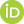## Publication Details

 Category Text Publication Reference Category Book chapters Title (Primary) Autocorrelation removal for spatial statistical modelling and its application to biogeographical data Title (Secondary) Wavelets: Classification, Theory and Applications Author Carl, G.; Kühn, I.Publisher del Valle, M.; Muñoz Guerrero, R.; Gutierrez Salgado, J.M. Year 2012 Department BZF Page From 67 Page To 95 Language englisch Abstract Biogeographical studies are often based on a statistical analysis of data sampled in a spatial context. If data sampled at adjacent locations are more likely to be similar to each other than distant ones, this is called spatial autocorrelation. However, in many cases standard analyses such as regression models may yield incorrect results, because the presence of autocorrelation violates the assumption of independently and identically distributed errors. First, we show that the theory of wavelets provides a method to remove autocorrelation in generalized linear models. Autocorrelation can be described by smooth wavelet coefficients at small scales. Therefore, data can be decomposed into uncorrelated and correlated parts. Using an appropriate linear transformation, we are able to extend generalized linear models to autocorrelated data. We illustrate our new method, called the wavelet-revised model, by applying it to multiple regressions with response variables conforming to various distributions. We recommend wavelet-revised models as a method for lattice data and applicable without any prior knowledge of the real autocorrelation structure. Second, we deal with the problem that statistical models may suffer not only from autocorrelation, but also from collinearity, i.e., predictor variables in a multiple linear regression can be highly correlated with each other. In the presence of collinearity among predictors, methods modelling, e.g., with latent variables such as partial least squares regression are recommended in literature. We introduce a novel approach coping simultaneously with both problems: collinearity and autocorrelation. Therefore, we develop an extension to partial least squares based on wavelet-revised modelling. We illustrate our new method, called wavelet-revised model for partial least squares (WRMPLS), by applying it to multiple regressions with responses of both normal and binary distribution. Its predictive performance is evaluated for simulated data. Persistent UFZ Identifier https://www.ufz.de/index.php?en=20939&ufzPublicationIdentifier=12372 Carl, G., Kühn, I. (2012): Autocorrelation removal for spatial statistical modelling and its application to biogeographical data In: del Valle, M., Muñoz Guerrero, R., Gutierrez Salgado, J.M. (eds.) Wavelets: Classification, Theory and Applications Nova Science Publishers, New York, p. 67 - 95